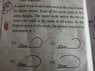# Normal Reaction in the following cases

• Kasul

## Homework Statement

A small block is shot into each of the four tracks as shown below. Each of the tracks rise to the same height. The speed with which the block enters the track is the same in all cases. At the highest point of the track, the normal Reaction is maximum in which track?

2. Homework Equations

At the highest point, N - mg = (mv^2)/r
So, N = (mv^2)/r + mg

## The Attempt at a Solution

I don't understand how the curvature of the track affects the normal reaction. I suppose it can't be option 3 and option 4 because those have greater radius and using the above mentioned formula, the normal reaction will be less. But why is the answer 1 when option 1 and 2 seem to have the same radius?

#### Attachments

•UC_Photo_001.jpg
58.6 KB · Views: 558

But why is the answer 1 when option 1 and 2 seem to have the same radius?
Fair question. They certainly look like the same radius, the only difference being that 1 continues further along the downward curve. Since that's beyond the highest point, it cannot affect anything at the highest point. The only other possibility is that the radii are not the same, they just look it to the unaided eye, but that would make it a poor question.

I'm sure it must have something to do with one track being extended but I don't know what difference that makes. Either that or the person that framed the question made a mistake.

I'm sure it must have something to do with one track being extended but I don't know what difference that makes. Either that or the person that framed the question made a mistake.
I'm certain it is a flawed question.

•Kasul

## Homework Statement

A small block is shot into each of the four tracks as shown below. Each of the tracks rise to the same height. The speed with which the block enters the track is the same in all cases. At the highest point of the track, the normal Reaction is maximum in which track?

2. Homework Equations

At the highest point, N - mg = (mv^2)/r
So, N = (mv^2)/r + mg

At the highest point, the direction of the centripetal force is downward, the resultant of gravity and the normal force, both downward. (mv^2)/r=N+mg, so N= (mv^2)/r - mg. So where can be the normal force maximum? At the smallest or greatest radius of curvature?

At the highest point, the direction of the centripetal force is downward, the resultant of gravity and the normal force, both downward. (mv^2)/r=N+mg, so N= (mv^2)/r - mg. So where can be the normal force maximum? At the smallest or greatest radius of curvature?
Are you suggesting that options 1 and 2 have different radii at their highest points? Doesn't look that way to me.

I suggested that the OP's equation for the normal force was wrong .
One peak of curves 1 and 2 looks a bit wider than the other one for me, but not considerably. I agree that it is a poorly presented question.